### Home > MC2 > Chapter 5 > Lesson 5.3.2 > Problem5-99

5-99.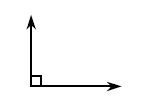The angle at right measures 90°. Estimate the measure (in degrees) of each angle below. Note: The angles are drawn to scale. Homework Help ✎

 a.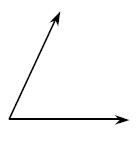b.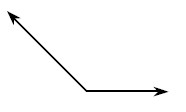c.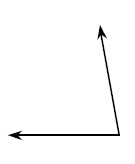d.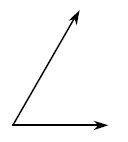e. If the angle in part (a) measures 65°, what would be the measure of its supplementary angle? Justify your answer.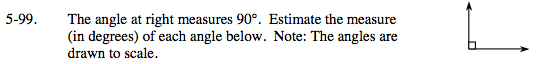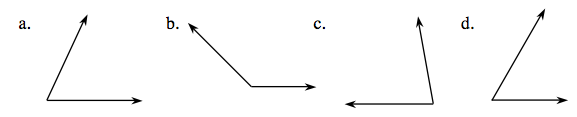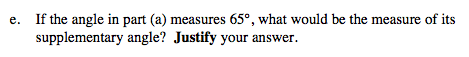Supplementary angles are angles that sum to make a 180° angle.

Use the slider in the eTool below to estimate the degree measurements for parts (a) - (d).
Click the link at right for the full version of the eTool: MC2 5-99 HW eTool